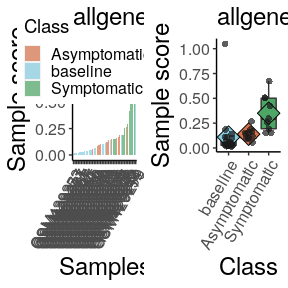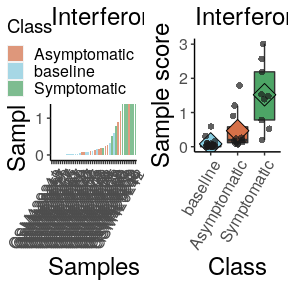# Running the mdp package

#### 2020-04-27

The Molecular Degree of Perturbation allows you to quantify the heterogeneity of transcriptome data samples. The mdp takes data containing at least two classes (control and test) and assigns a score to all samples based on how perturbed they are compared to the controls. Gene perturbation scores are calculated for each gene within each class. The algorithm is based on the Molecular Distance to Health which was first implemented in Pankla et al. 2009. It expands on this algorithm by adding the options to calculate the z-score using the modified z-score (using median absolute deviation), change the z-score zeroing threshold, and look at genes that are most perturbed in the test versus control classes.

## Basic usage

Load expression and pheno data and run:

library(mdp)
data(example_data) # expression data has gene names in the rows
data(example_pheno) # pheno data needs a Sample and Class column
mdp.results <- mdp(data=example_data, pdata=example_pheno, control_lab = "baseline")
#> Calculating Z score
#> Calculating gene scores
#> Calculating sample scores
#> printing
#> Warning: fun.y is deprecated. Use fun instead.

#> Warning: fun.y is deprecated. Use fun instead.### Sample scores

The sample scores can be accessed from the sample_scores element of the mdp results.

### Gene scores

For each gene in each class, a gene score is calculated, which is the average thresholded z-score value for that gene. A gene frequency is also calculated, which is the frequency that the gene is perturbed in a class.

gene_scores <- mdp.results$gene_scores gene_freq <- mdp.results$gene_freq
#>        Symbol  baseline Symptomatic Asymptomatic
#> HBA2     HBA2 0.1005193   0.5358329    0.0000000
#> HBA1     HBA1 0.1096699   0.2279062    0.0000000
#> ACTB     ACTB 0.2282284   0.5991647    0.2208631
#> UBB       UBB 0.1189312   0.0000000    0.0000000
#> HBB       HBB 0.1284082   0.3200307    0.0000000
#> IFITM2 IFITM2 0.0000000   0.0000000    0.0000000

### Perturbed genes

The mdp ranks genes according to the difference between their gene score in the test versus the control samples. The fraction_genes option for the mdp function allows you to control what top fraction of these ranked genes will count as the perturbed_genes. You can obtain a list of the perturbed genes from the mdp results,

perturbed_genes <- mdp.results$perturbed_genes ## Further usage ### Adding pathways Sample scores can also be calculated using genes that are within certain genesets. The mdp will accept genesets that are in the form of a list (see example below). You can read in a .gmt file of genesets using the fgsea::gmtPathways function from the fgsea package. file_address <- system.file("extdata", "ReactomePathways.gmt", package = "mdp") pathways <- fgsea::gmtPathways(file_address) mdp.results <- mdp(data=example_data, pdata=example_pheno, control_lab = "baseline",pathways=pathways) #> Calculating Z score #> Calculating gene scores #> Calculating sample scores #> printing #> Warning: fun.y is deprecated. Use fun instead. #> Warning: fun.y is deprecated. Use fun instead. #> Warning: fun.y is deprecated. Use fun instead. For each pathway, the signal-to-noise ratio of the test versus control sample scores will be calculated. You can access these results in the pathways element of the mdp results. head(mdp.results$pathways)
#>                                  Geneset Sig2noise
#> 2                         perturbedgenes 1.0065522
#> 7        Interferon alpha/beta signaling 0.8140508
#> 8                Interleukin-6 signaling 0.6002146
#> 3  Antigen processing-Cross presentation 0.5473415
#> 4                              Apoptosis 0.3819693
sample_scores <- mdp.results$sample_scores[["Interferon alpha/beta signaling"]] sample_plot(sample_scores,control_lab = "baseline", title="Interferon a/b") #> Warning: fun.y is deprecated. Use fun instead.### Z-score calculation options As a default, the mdp z-score normalises the expression data using the median as the averaging statistic. The standard deviation is estimated using the median absolute deviation mad function from the Stats package. If you would like to use the mean instead, select “mean”. mdp.results <- mdp(data=example_data, pdata=example_pheno, control_lab = "baseline", measure = "mean") #> Calculating Z score #> Calculating gene scores #> Calculating sample scores #> printing #> Warning: fun.y is deprecated. Use fun instead. #> Warning: fun.y is deprecated. Use fun instead. You can calculate the thresholded z-score using the compute_zscore function. A vector of control sample names must be provided. control_samples <- example_pheno[example_pheno$Class == "baseline","Sample"]
zscore <- compute_zscore(data = example_data,control_samples = control_samples,measure = "mean",std = 2)